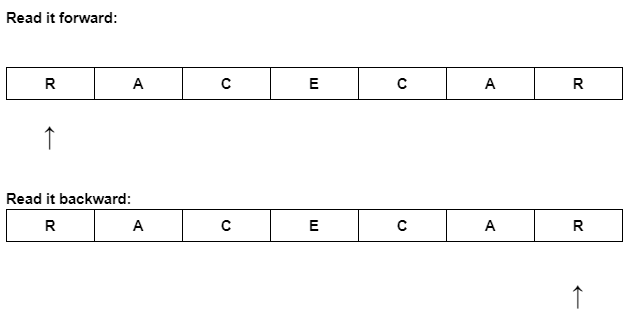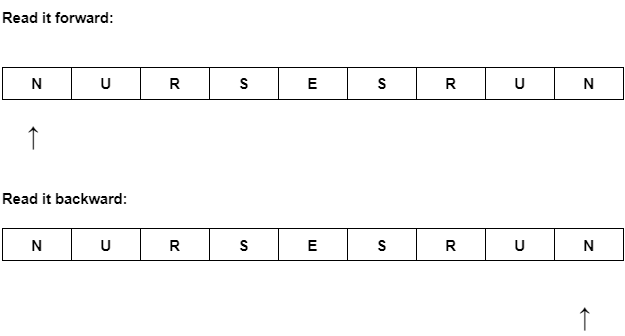×

Search anything:

# Generate all palindromes less than N

#### Algorithms List of Mathematical AlgorithmsGet this book -> Problems on Array: For Interviews and Competitive Programming

In this problem, we will see how to generate palindromic number in a given range efficiently by exploring the patterns of palindromes.

1 Introduction to problem
2 Approaches to solve a problem
3 Brute force solutions
4 Understanding the pattern of palindromic numbers
5 Optimized solution for generating palindromic numbers

### Introduction to problem

So, first of all, let us discuss what is a palindrome, palindrome is a sequence that reads the same backward as it read forward.

#### Example

Following are the examples of palindromes.

RACECAR

It will be read the same forward and backward illustrated below.Lets look at one more example.

NURSESRUN

It will also be read the same forward and backward illustrated below.So, you see palindrome sequence will be read the same no matter how you read it i.e. forward or backward.

The same goes for the numbers a number that is read the same backward as it is read forward is called a palindromic number.

#### Examples of palindromic number

Some palindromic numbers are given following.
11
101
1221
1123211

You can see no matter how you read the above number those would be read the same i.e. forward and backward.

### Approaches to solve a problem

There are 2 basic ways to solve any given problem
1 Find our solution by searching 1 by 1 in the whole dataset
2 Generate the relevant solution without searching into the dataset

### Brute froce solution

So, the first approach of almost every programmer to solve a problem is a brute force solution, so let's discuss the brute force solution to this problem.

The brute force solution to this problem is simply this.

#### Algorithm

1 start iterating from 1 up to the given number say N
2 call the number under iteration as original_number
3 reverse the original_number and call it reverse_number
4 compare the original_number and reverse_number
5 if both are equal print the original_number as palindrome
6 else increment the number which is under the loop by 1 and go to step 2 repeat it until the loop condition will be false

#### Python code

``````def palindrom_numbers(n):
for i in range(1,n):
original_number = str(i)
reverse_number = original_number[::-1]
if original_number == reverse_number:
print(original_number)

n = 12
palindrom_numbers(n)
``````

The time complexity of the above code is more than O(n) because the reverse string operation also takes additional time to convert a string into its reverse form and it depends upon the length of the number which is also variable.

So, this is the brute force solution in this solution our approach is basically to find the solution 1 by 1 in the whole dataset.

### Understanding the pattern of palindromic numbers

Now we will understand the pattern of palindromic solutions which will help us build the optimized solution for a problem. Let us again see some examples of palindromic numbers.
1
22
3333
1221
144441
131
11411
22322

If we categorize the above numbers based on length, we'll find out that there are two types of palindromic numbers exists i.e. odd length palindromic numbers(1, 131, 11411 ...) and even length palindromic numbers(22, 3333, 1221, ...).

#### Odd length palindromic numbers

If we try to understand the odd-length palindromic numbers we see a pattern in them the middle digit will be different and the digits on its right and left sides are mirrors of each other.
1 1 2 1 1
1 3 1
2 3 4 3 2

#### Even length palindromic numbers

Now try to understand what pattern exists in the even length palindromic numbers, in the even length palindromic numbers we see that the digits which are at the start of the number the mirror image of those digits are at the end of that number, the question is how we decide the end and start of even length number the answer is quite simple to divide the number into two parts on the base of its length the first part will be the start and the second part will be the end of the number. Let's look at the following examples.
2 2
3 3 3 3
1 2 2 1

All the above numbers have even lengths let's discuss the above numbers 1 by 1.

• In 22 the length of the number is two if we divide it into two parts the length of each part would be one digit so, 2 is at the start of the number as well as at the end of the number representing the mirror of starting digit.
• In 3333 both parts i.e. start and end of numbers would contain two digits, so 33 is at the start of the number and its mirror which is also 33 is at end of the number.
• In 1221 both parts of numbers contain two digits, the start part has 12, and the end part has its mirror 21 now see in this example the pattern of even length palindromic numbers is explained very well.

All even-length palindromic numbers have the same pattern explained above.

### Optimized solution for generating palindromic numbers

Now we understand the pattern of palindromic numbers, so we can build an optimized solution to the given problem. So, now we know that there are two types of palindromic i.e. even length and odd length numbers that exist in any given range. So, if we think a bit we'll be able to come up with an algorithm that can generate both types of palindromic numbers up to a given range.

Let's say we have to generate all palindromic numbers which are less than 104.

#### Algorithm

1 Call the given number n(104 in our case)
2 Start iterating from number 1 and call it i
3 use i to generate the even length palindrome(with some mathematical formula) and call it pal
4 print the pal
5 increment i by 1
6 go to step 3 and repeat the process until the pal is less than n.

Use the above algorithm once more to generate the odd length palindromes(i.e. in step 3 of the above algorithm generate odd length palindrome).

When the above algorithm will be completed all the palindromes have been generated which are less than a given number(104).

Now for the sake of making everything understandable let me code the above algorithm in two parts, one part will generate all even length palindromes and the other part will generate all odd length palindromes, in the end, I'll merge these two parts and devise a single code which will generate all the palindromes less than the given range.

#### Code for even length palindromes

``````def generate_even_length_palindromes(i):
n = i
while( n > 0):
i = i * 10 + (n % 10)
n = n // 10
return i
``````

Lets dry run our above code for a number 12

• Initially n = 12
• Our condition will be true and i will be 12 * 10 + (2) = 122
• Now n will be 1 after execution of this statement n = n // 10
• Still condition is true and i will be 122 * 10 + (1) = 1221
• Now n will be 0 after execution of this statement n = n // 10
• Condition will be false now the i will be returned from the function which will be 1221 which is an even length palindrome.

This is how the above function will work to generate even length palindromes you can dry run it or execute it for any number.

#### Code for odd length palindromes

``````def generate_odd_length_palindromes(i):
n = i // 10
while( n > 0):
i = i * 10 + (n % 10)
n = n // 10
return i
``````

Lets dry run our above code for a number 12

• Initially n = 1
• Our condition will be true and i will be 12 * 10 + (1) = 121
• Now n will be 0 after execution of this statement n = n // 10
• The condition will be false now the i will be returned from the function which will be 121 which is an odd length palindrome.

This is how the above function will work to generate odd length palindromes you can dry run it or execute it for any number.

#### Complete code for generating all palindromes less than N

``````def generate_palindromes(i, is_odd):
# logic/ mathematical formula
# to generate palindromes
if (is_odd):
n = i // 10
else:
n = i
while(n > 0):
i = i * 10 + (n % 10)
n = n // 10
return i

for k in range(2):
# this loop has only two iterations,
# 1st to generate even length
# 2nd to generate ood length
i = 1
while(generate_palindromes(i, k%2) < 100000):
# iterate and generate a palindrom until
# it greater than the given range
print(generate_palindromes(i,k%2))
i += 1

``````

Above is the completed code to generate all the palindromes within a given range. It's a product of the above two functions(generate_odd_length_palindromes, generate_odd_length_palindromes) which I have explained to make you understand the whole logic of solving this problem.#### Ali Haider

Currently working as a Software Engineer - WEB in a Fintech company named as i2c Inc. I am continuously striving for best. Completed my BS Computer Science degree from University of Gujrat Pakistan.

Generate all palindromes less than N